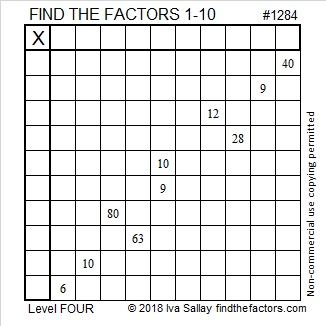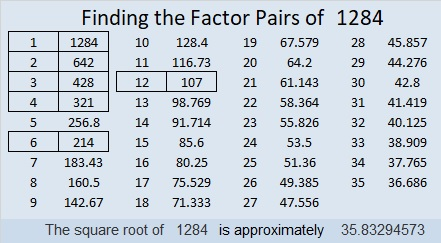# 1284 and level 4

Ten clues are in this puzzle. Two of them are 9’s and two of them are 10’s, but that doesn’t cause any problems, . . . .probably!Print the puzzles or type the solution in this excel file: 10-factors-1281-1288

Here are some facts about the number 1284:

• 1284 is a composite number.
• Prime factorization: 1284 = 2 × 2 × 3 × 107, which can be written 1284 = 2² × 3 × 107
• The exponents in the prime factorization are 2, 1, and 1. Adding one to each and multiplying we get (2 + 1)(1 + 1)(1 + 1) = 3 × 2 × 2 = 12. Therefore 1284 has exactly 12 factors.
• Factors of 1284: 1, 2, 3, 4, 6, 12, 107, 214, 321, 428, 642, 1284
• Factor pairs: 1284 = 1 × 1284, 2 × 642, 3 × 428, 4 × 321, 6 × 214, or 12 × 107
• Taking the factor pair with the largest square number factor, we get √1284 = (√4)(√321) = 2√321 ≈ 35.832951284 is the sum of twin primes: 641 + 643 = 1284

This site uses Akismet to reduce spam. Learn how your comment data is processed.